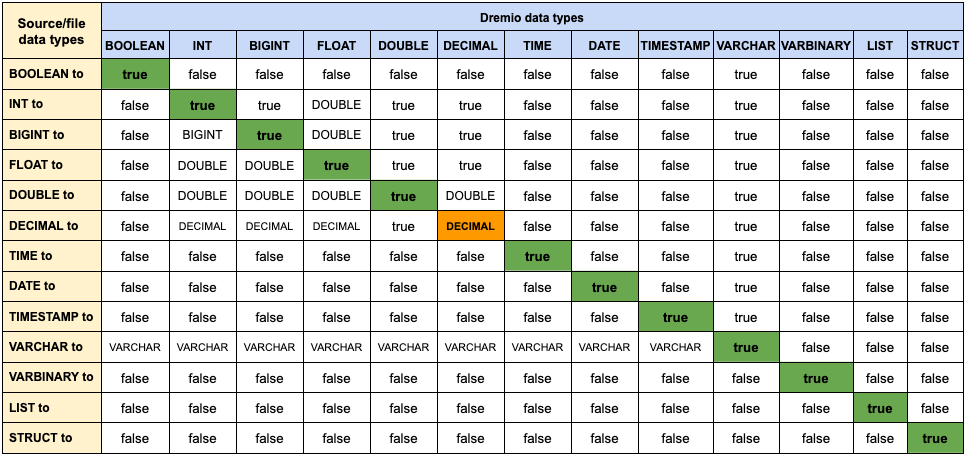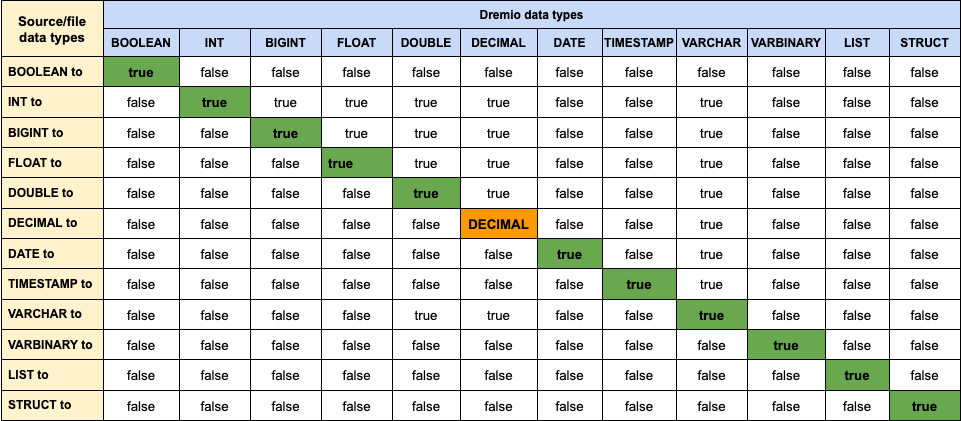# Data Types

## Supported Data Types

Data TypeUI SymbolMaximum Size
BOOLEANNA
VARBINARY32000 bytes
DATENA
FLOATNA
DECIMAL*NA
DOUBLENA
INTERVAL (day to seconds)NA
INTERVAL (years to months)NA
INTNA
BIGINTNA
TIMENA
TIMESTAMPNA
VARCHAR**32000 bytes
STRUCTNA
LISTNA

* Partially supported
** _`VARCHAR` type only supports UTF-8 encoded values_

### `LIST` and `STRUCT` Literals

Dremio does not have `LIST` or `STRUCT` literals, but you can get the same result using `CONVERT_FROM` and JSON strings:

``````SELECT CONVERT_FROM('["apple", "strawberry", "banana"]', 'json')
-- ["apple","strawberry","banana"]

SELECT CONVERT_FROM('{"name":"Gnarly", "age":7, "car":null}', 'json')
-- {"name:"Gnarly","age":7}
``````

### Decimal Support

As of Dremio 4.0, decimal to decimal mappings are supported for relational database sources. That is, when creating a new relational database data source, the default is to map decimal to decimal. If you do not want the default decimal-to-decimal mapping, you can enable decimal-to-double mappings through the data source Advanced Options tab.

Note:

Upgrade Impact: When you upgrade a relational data source to Dremio 4.0 with decimal usage, the existing (pre-4.0) behavior of mapping decimals to doubles is retained.

Dremio fully supports Decimal data type for Parquet, Hive(Parquet/ORC) sources. Text/Json sources can cast strings/integers to decimals for decimal precision and scale. Other sources treat decimals as doubles same as prior to 3.3.5.

#### Return Type Inference

The return type inference provides the return type for functions and operators that natively handle decimal types. Functions and operators that downcast instead return the data type in the existing function (double/float/int).

Dremio supports the following return type inference rules:

##### Arithmetic Operators
Function/OperatorNative Decimal Support
- (Subtraction)
s = max(s1, s2)
p = max(s1, s2) + max(p1 - s1, p2 - s2) + 1

```if (p > 38) { minS = min(s, 6) s = s - (p - 38) s = max(minS, s) P = 38 }```
* (Multiplication)s = s1 + s2
p = p1 + p2 + 1

```if (p > 38) { minS = min(s, 6) s = s - (p - 38) s = max(minS, s) p = 38 }```
/ (Division)s = max(6, s1 + p2 + 1)
p = p1 -s1 + s2 + s

```if (p > 38) { minS = min(s, 6) s = s - (p - 38) s = max(minS, s) p = 38 }```
% (Modulus)s = max (s1, s2)
p = min (p1 - s1, p2 - s2) + s

```if (p > 38) { minS = min(s, 6) s = s - (p - 38) s = max(minS, s) p = 38 }```
##### Mixed Types
Function/OperatorNative Decimal Support
Mixed Typess = max(s1, s2)
p = max(s1, s2) + max(p1 - s1, p2 - s2)

```if (p > 38) { Error out and reject. }```
##### SQL Clauses
Function/OperatorNative Decimal Support
ORDER BYSame as input precision and scale
GROUP BYSame as input precision and scale
##### Math Functions
Function/OperatorNative Decimal Support
ABS()Same as input precision and scale
CEILING()Same as input precision and scale
FLOOR()Same as input precision and scale
MOD()Same as Modulus above
ROUND()Same as input precision and scale
TRUNC()Same as input precision and scale
##### Aggregate Functions
Function/OperatorNative Decimal Support
AVG()Decimal(38,s) / Decimal(18,0)
MAX()Same as input precision and scale
MIN()Same as input precision and scale
SUM()Decimal(38, input Scale)
##### Analytic Functions
Function/OperatorNative Decimal Support
LAG()Same as input precision and scale
LEAD()Same as input precision and scale
##### Type Conversion Functions
Function/OperatorNative Decimal Support
CAST()Provided in cast type
CONVERT_FROM())Provided in cast type
CONVERT_TO()Provided in cast type
##### Set Operators
Function/OperatorNative Decimal Support
UNION()TBD
UNION ALL()TBD
INTERSET()TBD
MINUS()TBD

#### Mixed Decimal Types

The following decimal union types specifies when mixed decimal types are supported:

• Parquet – Mixed decimal types in a data set are not supported. For example, the same column cannot have differing precision/scale across files in the same dataset.
• ORC – Mixed decimal types in a data set are supported.

#### Decimal Limitations

The following are decimal limitations:

• Decimal numeric literals in SQL queries cannot be larger than maximum possible long value.

• Queries that do arithmetic on a column and literal have issues.

For example, `select cast(12345 as double) * CAST(A as double)` fails. The work around is to use a string value such as `select cast('12345' as double) * CAST(A as double)`.

• Queries casting numeric literals to decimal should use specific precision. Precision of literal cannot be lowered.

For example, `cast(123.23 as decimal(2,0))` returns the same number as output since given number cannot be represented using precision 2.

• In general, decimal overflow is not handled; output when decimal arithmetic overflows is undefined. This is the same as other data types in Dremio.

### Timestamp Queries

When Dremio retrieves the timestamp value entry in a table, the returned timestamp entry is displayed as it was written to the file system. That is, Dremio does not apply any conversions to the timestamp entry.

For example:

• If the timestamp value entry is written to the file system in UTC, then Dremio displays the entry in UTC.
• With Hive, timestamp value entries are converted and stored as UTC in the file system.
Subsequently, when Dremio returns the timestamp value entry, it is displayed in UTC.
• With some other application, if the timestamp value entry is written and stored as PST (local time) in the file system, then when Dremio returns the timestamp value entry, it is displayed in PST.

## Supported Implicit Coercions/Conversions for File-formatted Data Types

If the data type found within a source file does not match the data type for the table, one of the following actions is taken:

1. During a read, Dremio performs an implicit coercion/conversion from the source file data type to the table data type so it matches the data type defined in Dremio. After coercion all SQL processing is performed using the table’s defined schema and data types.
2. Dremio automatically updates the table’s data type to incorporate the new data types found and then reruns the query, a process called schema learning.
3. If the data types cannot be coerced due to belonging to a different data type family, the query fails to run and you see an error message.

For metastore sources such as Apache Hive and AWS Glue, Dremio does not update the data type since the table’s schema is defined by the external metastore. Additionally, for Apache Iceberg and Delta Lakes, the table format defines the schema and there is no schema learning.

The following Supported and Unsupported Coercions tables show the data type coercions supported in Dremio and when implicit coercion/conversion can or cannot be performed for file-formatted, AWS Glue, and Apache Hive sources.

Notes about the Supported and Unsupported Coercions tables:

• The header row represents the data type in Dremio.
• The first vertical column represents the data type found in an external source.
• The value in a cell identifies the action for the data type:
• True: The data type from the source file is successfully coerced to the Dremio data type.
• Data type indicator: The data type for the column is automatically updated to the stated data type, and the query is rerun. For example, if a column is originally defined as an INT type and Dremio encounters a BIGINT, Dremio will update the data type to BIGINT through schema learning.
• False: No coercion is available and an error is returned.
• VARCHAR: represents STRING/VARCHAR
• For additional information about the DECIMAL-to-DECIMAL conversion shown in the tables, see DECIMAL-to-DECIMAL Coercions

Note:

These tables will be updated as additional implicit conversions become available.

Table 1: Supported and Unsupported Coercions for File-formatted SourcesTable 2: Supported and Unsupported Coercions for Apache Hive ORC DatasetsFor Apache Hive and AWS Glue sources with Parquet datasets, Dremio supports the following coercions:

• INT to BIGINT
• FLOAT to DOUBLE
• DECIMAL to DECIMAL
• VARCHAR to VARCHAR[N], which truncates VARCHAR to the specified [N] limit

### DECIMAL-to-DECIMAL Coercions

Since decimals can have different scales and precision, Dremio supports DECIMAL-to-DECIMAL coercions. Decimal coercion support is provided in the following DECIMAL-to-DECIMAL Overflow/Truncation Behavior table.

Note:

The following symbols are used in the DECIMAL-to-DECIMAL Overflow/Truncation table:

• Precision (p): represents the number of digits in the value/cell.
• Scale (s): represents the number of digits after the decimal point in the value/cell.

Table 3: DECIMAL-to-DECIMAL Overflow/Truncation Behavior

Source/File Data TypeTable SchemaDescriptionOn OverflowOn Truncation
DecimalDecimal[Conversion between any DECIMAL type is supported, including decreasing the `p` or `s`. Checks that are added during runtime help to detect overflow.NullRounds up to the nearest half

Note:

Implicit conversions/coercions are not currently supported for filesystem and MongoDB sources.

### DECIMAL-to-DECIMAL Schema Learning

When source files contain decimal values using a different scale or precision from the table’s definition - `Decimal (p, s)` - Dremio will update the table’s decimal definition according to the following logic through schema learning if possible, or otherwise attempt to coerce the source decimal values to the table’s definition.

Logic used for the schema learning option, where `d` = decimal, `p` = precision, and `s` = scale:

• `d1`, `p1`, `s1` - The decimal type in file 1 with a given `p` and `s`
• `d2`, `p2`, `s2` - The decimal type in file 2 with a given `p` and `s`
• Precision - The new precision after schema learning
• Scale - The new scale after schema learning

The following shows the schema-learned decimal type for the column:

• `Precision = max(s1, s2) + max(p1-s1, p2-s2)`

Note:

This schema provides space for the maximum fractional part (below decimal point) and integral part (above decimal point).

• `Scale = max(s1, s2)`

If the resulting precision is greater than 38, it is reduced to 38, and the corresponding scale is reduced to prevent truncating the integral part:

• Scale is reduced to provide enough space for the integral part. The resulting scale is `MIN(precision, 38) - max(p1 - s1, p2 - s2)`. The resulting precision is 38.
• This result causes truncation on read since the fractional part will not contain enough spaces for the original scale.

## Source Data Type Mappings

The following topics provide Dremio data type mappings to source database types: